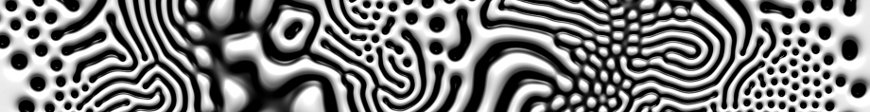Reaction-Diffusion Tutorial Karl Sims A simulation of two virtual chemicals reacting and diffusing on a 2D grid using the Gray-Scott model

 Chemical A is addedat a given "feed" rate. Chemical B is removedat a given "kill" rate.Reaction: two Bs convert an A into B, as if B reproduces using A as food.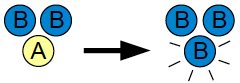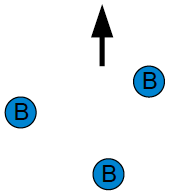Diffusion: both chemicals diffuse so uneven concentrations spread out across the grid, but A diffuses faster than B. The system is approximated by using two numbers at each grid cell for the local concentrations of A and B. (The particles are not individually simulated.)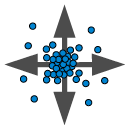When a grid of thousands of cells is simulated, larger scale patterns can emerge.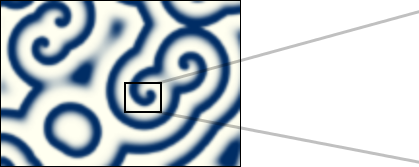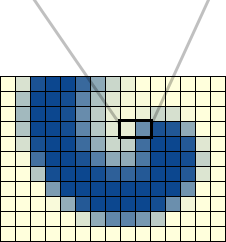The grid is repeatedly updated using the following equations to update the concentrations of A and B in each cell, and model the behaviors described above.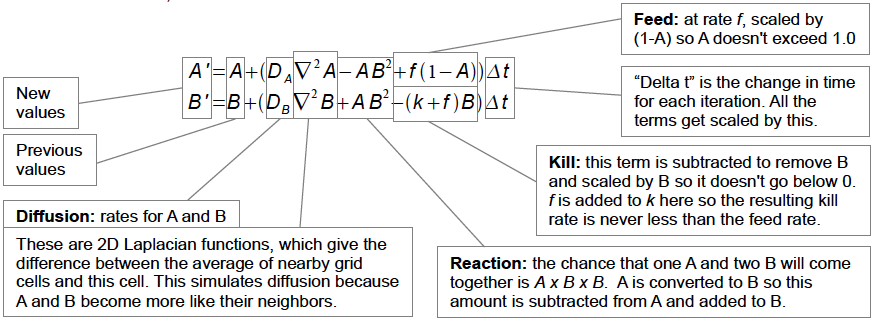Some typical values used, for those interested, are: DA=1.0, DB=.5, f=.055, k=.062 (f and k vary for different patterns), and Δt=1.0. The Laplacian is performed with a 3x3 convolution with center weight -1, adjacent neighbors .2, and diagonals .05. The grid is initialized with A=1, B=0, and a small area is seeded with B=1.

Surprisingly complex and dynamic behaviors can arise from these fairly simple rules, and adjusting the two parameters for the feed rate and kill rate can produce a range of different results. The grid is visualized by assigning each cell a color from it's A and B values. Here A is white and B is black.The reaction naturally has two stable states: lots of A with no B to consume it, or lots of B where new A is quickly converted into more B. But the diffusion can cause interesting behaviors at the borders between the mostly-A and mostly-B areas. In this "mitosis" example, at convex borders more A is available nearby to diffuse inwards and feed B, so those edges grow outwards as B increases and diffuses. At concave borders, less A diffuses in and B slowly thins out and dies off. This can cause a spot of B to grow and then divide into two as if it were a cell undergoing mitosis.Here are two videos that show a "mitosis" simulation (f=.0367, k=.0649) and a "coral growth" simulation (f=.0545, k=.062). In these examples, a lighting model is also used to give the shapes a 3D embossed look.

Additional options can give more possible effects:
Orientation: diffusion can occur faster in one direction than another to give an orientation to the results.
Style Map: the feed and kill rates can vary across the grid to give different patterns in different areas.
Flow: the chemicals can flow across the grid to give various dynamic effects.
Scale: the size of the pattern changes when the reaction rate is sped up or slowed down relative to the diffusion rate.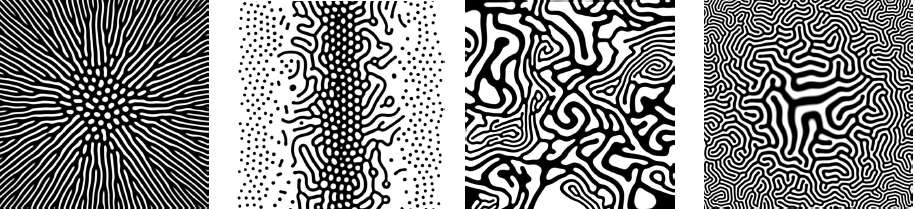The map below on the left shows the resulting patterns when the kill rate varies along the x axis (from .045 to .07) and the feed rate varies along the y axis (from .01 to .1). Some areas are stable with solid A or solid B, but a crescent shaped zone between these gives various types of complex behaviors. The second image is a transformed version of this map. The more interesting areas have been expanded and warped to fall within a rectangular shape, which can be useful as a user-interface for selecting pairs of k,f values to generate different reaction-diffusion patterns.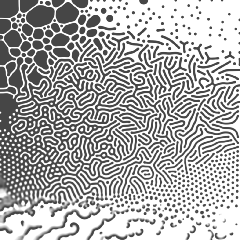For further information

Try this RD Tool web application to experiment with reaction-diffusion simulations.

Visit the Reaction-Diffusion Media Wall exhibit at the Museum of Science, Boston.